Home MonkeyNotes Printable Notes Digital Library Study Guides Study Smart Parents Tips College Planning Test Prep Fun Zone Help / FAQ How to Cite New Title Request

2.6 Evaluating Limits

Limits of functions are evaluated by using many different methods ( techniques ) such as recognizing a type, simple substitution, factorization, simplification, rationalization etc. Let us solve a few examples by using each of these methods.

1.  Substitution

Example 1 Evaluate lim ( 5x2 - 7x + 9 )
x®3

Solution : Let L = lim ( 5x2- 7x + 9 )
x®3

= 5 (3)2 - 7 (3) + 9 \ x ¹ 3

= 33

i.e. when x is replaced by 3, (5x2 - 7x + 9 ) ® 33

Hence lim ( 5x2 - 7x + 9 ) = 33
x® 3

2. Factorization

Example 2  Evaluate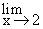Solution : Let L =Direct substitution of x = 2 yields 0/0 form which means less. By Factoring, simplifying and then substituting we find that

L ==( x + 2 )

= 2 + 2x ¹ 2

= 4

Note that the graph of y   =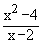is the same as the graph of y = ( x + 2 ) except it has a break at (2,4)Your browser does not support the IFRAME tag.

Example 3  Evaluate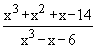Solution :  Let  L =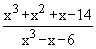Substituting x = 2 yields 0/0 form which is meaning less. Hence ( x - 2 ) must be a factor of both numerator and denominator. Let us factorize them by synthetic division.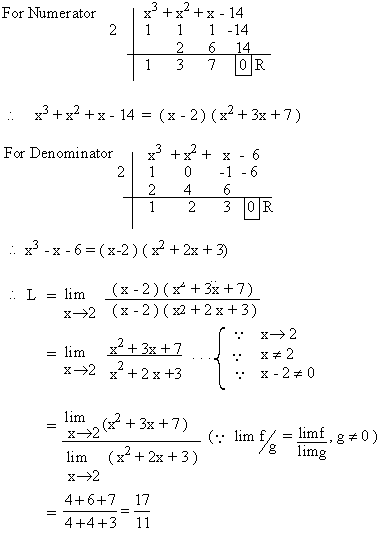Index

2.1 Modulus
2.2 Inequalities
2.3 Limits Of Functions
2.4 Left Hand And Right Hand Limits
2.5 Theorems On The Algebra Of Limits
2.6 Evaluating Limits
2.7 Limits Of Trigonometric Functions
2.8 The Exponential Limits

Chapter 3